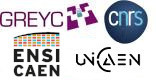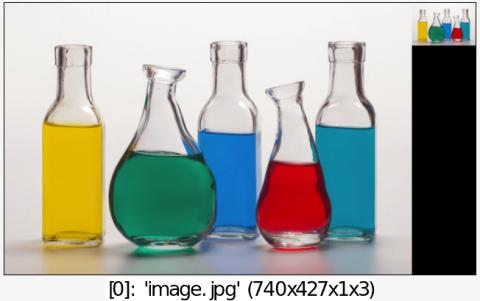G'MIC - GREYC's Magic for Image Computing: A Full-Featured Open-Source Framework for Image Processing# A Full-Featured Open-Source Framework for Image Processing

## Latest stable version: 3.1.5

Reference

 Table of Contents  ▸  List of Commands  ▸  Geometry Manipulation  ▸  resize2din ◀  resize_ratio2d    |    resize3din  ▶

# resize2din

## Arguments:

• width[%]>0,_height[%]>0,_interpolation,_boundary_conditions,_ax,_ay,_az,_ac

## Description:

Resize selected images so the size is not larger than width'x'height while preserving 2D ratio.

(equivalent to shortcut command r2din).

interpolation can be { -1=none (memory content) | 0=none | 1=nearest | 2=average | 3=linear | 4=grid | 5=bicubic | 6=lanczos }.
boundary_conditions has different meanings, according to the chosen interpolation mode :
. When 'interpolation=={ -1 | 1 | 2 | 4 }', boundary_conditions is meaningless.
. When interpolation==0, boundary_conditions can be { 0=dirichlet | 1=neumann | 2=periodic | 3=mirror }.
. When 'interpolation=={ 3 | 5 | 6 }', boundary_conditions can be { 0=none | 1=neumann }.
ax,ay,az,ac set the centering along each axis when interpolation=0
(set to 0 by default, must be defined in range [0,1]).

## Default values:

height=100%, interpolation=3, boundary_conditions=0 and ax=ay=az=ac=0.

## Example of use:

\$ gmic image.jpg +resize2din 100,100 append xCommand: image.jpg +resize2din 100,100 append x
G'MIC - GREYC's Magic for Image Computing: A Full-Featured Open-Source Framework for Image Processing